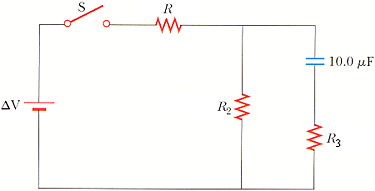Ask question

# Find the length of the leg of the triangle 45-45-90 with a long hypotenuse of 25 inches.# Find the length of the leg of the triangle 45-45-90 with a long hypotenuse of 25 inches.

Question
Right triangles and trigonometryasked 2021-02-24
Find the length of the leg of the triangle 45-45-90 with a long hypotenuse of 25 inches.

## Answers (1)2021-02-25
In a 45°-90°90° triangle, the length of the hypotenuse is 2–sqrt2 times each leg.
If x is the leg length, then we can write:
$$25=\sqrt{2\times x}$$
$$\frac{25}{\sqrt 2}= x$$
$$\frac{25}{\sqrt 2}\times \frac{\sqrt 2}{\sqrt 2}$$
$$x=\frac{(25\times \sqrt2)}{2}$$

### Relevant Questionsasked 2020-11-07
In quadrant I, drawn a right triangle with one leg on the x-axis and its hypotenuse on the terminal side of drawn in standard position. If sin = 7/25, then what is the value of theta θ?asked 2021-01-25

In $$\triangle PQR$$, identify the hypotenuse, adjacent leg, and opposite leg for $$\angle R$$.asked 2020-11-26
Find the length of a leg of an isosceles right triangle whose hypotenuse has length 12asked 2020-10-18
Use function notation to describe the way the second variable (DV) depends upon the first variable (IV). Determine the domain and range for each, determine if there is a positive, negative, or no relationship, and explain your answers.
A)IV: an acute angle V in a right triangle: DV: the area B of the triangle if the hypotenuse is a fixed length G.
B)IV: one leg P of a right triangle: DV: the hypotenuse G of the right triangle if the other leg is 2
C)IV: the hypotenuse G of a right triangle: DV: the other leg P of the right triangle is one leg is 5.asked 2020-12-13
Expanding isosceles triangle The legs of an isosceles right tri- angle increase in length at a rate of 2 m/s.
a. At what rate is the area of the triangle changing when the legs are 2 m long?
b. At what rate is the area of the triangle changing when the hypot- enuse is 1 m long?
c. At what rate is the length of the hypotenuse changing?asked 2021-02-09
what is the length of the hypotenuse of a right triangle with legs of length 7 and 8asked 2021-01-16
The length of the base of an isosceles triangle is x. The length of a leg is 2x - 6. The perimeter is 53. Find x.asked 2021-03-21
In the figure below, suppose that the switch has been closed for a length of time sufficiently long enough for the capacitor to become fully charged. (R = 13.0 k, R2 = 20.0 k, R3 = 5.00 k, ΔV = 9.30 V)(a) Find the steady-state current in each resistor.
(b) Find the charge on the capacitor.asked 2021-02-14

Find the probability of the indicated event if $$P(E)=0.25\ and\ P(F)=0.45. P( E\ or\ F ) if E$$ and Fare mutually exclusiveasked 2021-01-31
Two right triangles are similar. The legs of the smaller triangle have lengths of 3 and 4. The scale factor is 1:3. Find the length of the hypotenuse of the larger triangle.
...